# Determinants - Online Test

Q1. Let A =, then Det. A is
Explaination / Solution: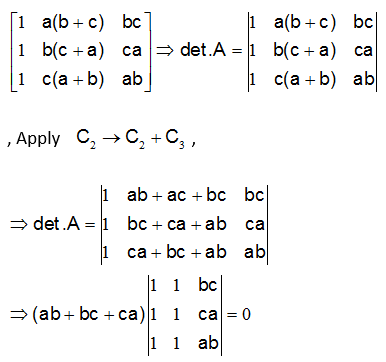( since C1 and C2 are identical)

Q2.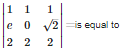Explaination / Solution:because , row 1 and row 3 are identical.

Q3. If A, B be two square matrices such that |AB|=O, then
Explaination / Solution:

If A B be two square matrices such that AB=O, then, |AB|=O⇒|A||B|=O⇒either|A|=0,or|B|=0

Q4. A square matrix A is invertible iff det A is equal to
Explaination / Solution:

Only non-singular matrices possess inverse.

Q5. If A and B are square matrices of order 3 , then
Explaination / Solution:

If A B be two square matrices of order 3 and if |B|=0, AB=0 then this is possible only if |A| may be zero

Q6. The value of the determinantExplaination / Solution: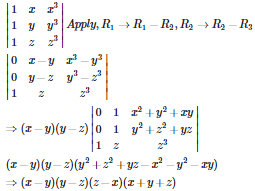Q7. One root of the equation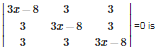Explaination / Solution: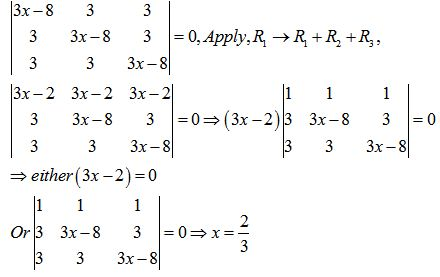Q8.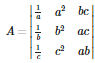is equal to
Explaination / Solution: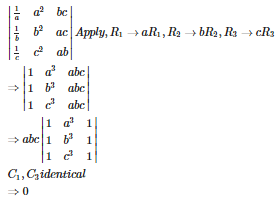Q9. If A ,B andC be the three square matrices such that A = B + C , then Det A is equal to
Explaination / Solution:

Because , if A=B+C then |A|=|B+C|

Q10. If  is non real cube root of unity , then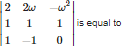Explaination / Solution:

expanding along R